Next: SR Spectroscopy Up: SR Studies of the Previous: List of Figures   Contents

# Introduction

In 1986, Bednorz and Müller discovered superconductivity in the La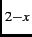Ba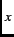CuO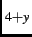system, below a critical transition temperature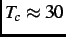K . This finding was subsequently followed by the discovery of high-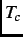superconductivity in a number of related layered compounds consisting of CuO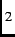planes. The CuOplanes in these materials are separated by charge reservoir layers'', which control the oxidation state of the copper ions. High-superconductivity is achieved when the undoped parent compound (e.g. LaCuO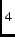), which is an antiferromagnetic insulator, is doped with holes (Fig. 1.1) . An exception is the RCeCuOsystem, where R = {La, Nd, Pr, Sm or Eu}. In this case, high-superconductivity occurs upon partial substitution of Ce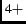for R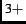, which dopes electrons into the CuOlayers. The most studied electron-doped high-superconductor (HTSC) is NdCeCuO, which was synthesized by Tokura and co-workers in 1989 . Compared to hole-doped HTSC's, less is known about the electron-doped cuprates. One reason is that it is difficult to synthesize high-quality single crystals. In contrast to the hole-doped compounds, as-grown crystals are made superconducting by subjecting them to an oxygen reduction process , which often degrades the crystal surface. In addition, single crystals of electron-doped cuprates are generally plagued by spatial inhomogeneity of the cerium and oxygen concentration [5,6].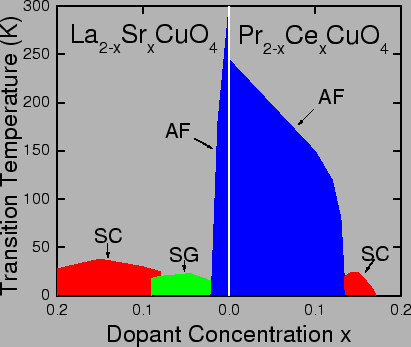One of the fundamental properties of superconductors is that they exhibit a diamagnetic response called the Meissner effect.'' When a superconducting material is cooled belowin the presence of a weak applied magnetic field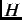, the field is expelled from the bulk of the sample. When the applied magnetic field exceeds a critical value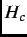, superconductivity is destroyed. For type-II superconductors, like the high-cuprates, the response to an applied magnetic field can be one of the following:

1. Meissner State: At<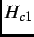, (where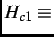the lower critical magnetic field), supercurrents circulate near the surface of the sample, screening the magnetic field from the bulk. However, the external magnetic field partially penetrates the sample at the surface. The magnitude of the penetrating field-component parallel to the surface decays exponentially  as a function of distance from the surface. The characteristic length scale of the exponential field decay is the magnetic penetration depth''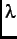. This quantity is of fundamental importance, as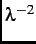is proportional to the density of superconducting carriers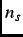.

2. Vortex State: At<<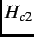(where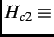the upper critical magnetic field), magnetic flux penetrates the bulk of the superconductor in the form of a periodic arrangement of quantized flux lines, called a vortex lattice''. Each vortex in the lattice is comprised of one flux quantum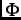=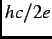. The vortex core is a region where the superconducting order parameter''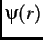is suppressed and the local magnetic field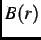is maximum (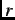is the radial distance from the centre of the vortex). The length scale which governs spatial variations ofis called the superconducting coherence length''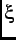. Supercurrents circulating around the individual vortices screen the magnetic field within the vortex core from the surrounding material, in the same way that supercurrents near the sample surface screen the external magnetic field in the Meissner state. Consequently, the magnetic field decays outside the vortex core region over the length scale.

3. Normal State:>, the external magnetic field fully penetrates the sample and superconductivity is destroyed.

One of the outstanding issues concerning the electron-doped HTSCs is the pairing symmetry of the superconducting carriers in these compounds. While there are phase sensitive , angle resolved photoemission spectroscopy  and microwave  measurements that suggest the pairing symmetry is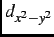-wave (like in hole-doped HTSCs), other experiments [11,12] favour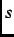-wave symmetry. Measurements of the temperature dependence of the magnetic penetration depth are one way of distinguishing between-wave and-wave symmetry. Thus far, most measurements ofin electron-doped HTSCs have been performed in the Meissner state. In this thesis, the results of measurements of the in-plane magnetic penetration depth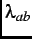in the vortex state of the electron-doped HTSC PrCeCuO, by muon spin rotation (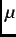SR) spectroscopy are presented. As a bulk local probe,SR has the advantage that it is insensitive to inhomogeneities at the sample surface. The samples measured are the smallest single crystals studied so far by theSR techniques.A complication in studying electron-doped HTSCs, is the electronic magnetic moments of the rare-earth ions. For example, their presence has prevented an accurate determination of the values of the magnetic penetration depth in the Meissner state [13,14]. The PrCeCuOcompound is appealing for study, because the crystal-electric-field ground state of the Pr ion is non-magnetic.

In the next chapter an introduction to theSR method is given. Chapter 3 provides a brief description of the crystal growth process, sample characteristics and the experimental setup. In Chapters 4 and 5, the experimental results are presented. A discussion of the results and conclusions are given in Chapter 6.Next: SR Spectroscopy Up: SR Studies of the Previous: List of Figures   Contents
Jess H. Brewer 2003-07-01